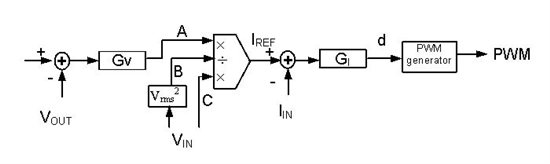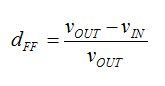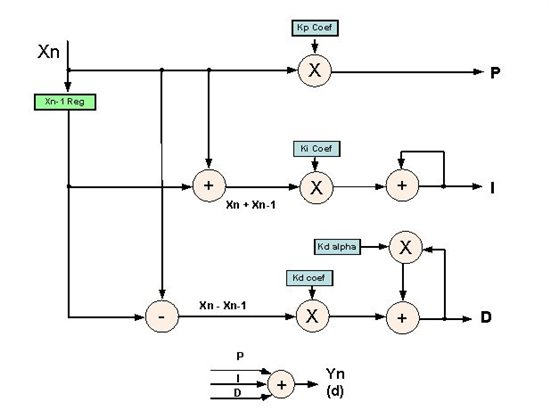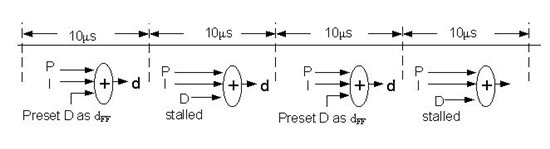# How To: Improve power factor and THD using DFF control

For decades, average current-mode control has been used in power factor correction (PFC), and various PFC control chips that employ this control algorithm can be found in the commercial market.

Figure 1 depicts this average current-mode control.Figure 1. Average current-mode control for PFC.

The performance of average current-mode control is often considered adequate for most commercial power applications with 50/60 Hz AC line input. However, the traditional average current-mode control causes the inductor current to lead the input voltage, resulting in a non-unity fundamental displacement power factor and zero-crossing distortion. This situation gets worse when the PFC operates in a high-frequency AC environment, such as an airborne system which operates at 400 Hz. The required high quality of the input current needed for these systems is difficult to achieve through traditional control method. A new control method called duty ratio feedforward (DFF) control can effectively reduce input current distortion under high line frequencies 1/2/3.

The basic idea of DFF control is to pre-calculate a duty ratio to alleviate the task of the feedback controller. For the boost topology operating in continuous conduction mode, the duty ratio dFF is given by:Equation 1.

This duty ratio pattern produces a voltage across the switch whose average over a switching cycle is equal to the rectified input voltage. A regular current loop compensator changes the duty ratio around this calculated duty ratio pattern.

Since the impedance of the boost inductor at the line frequency is very low, a small variation of the duty ratio produces enough voltage across the inductor to generate the required sinusoidal current waveform.Figure 2. Duty ratio feedforward control for PFC.

Figure 2 depicts the resulting control scheme. Equation 1 calculates the feedforward duty ratio dFF. It is then added to the traditional average current-mode control output dI. The final duty ration d is used to generate a pulse-width modulated (PWM) waveform to control PFC.

When using DFF control, you need many mathematical calculations. The CPU's speed determines the control loop speed, which then impacts the loop bandwidth. A faster CPU means achieving a higher bandwidth. However, it also means more expensive and higher power consumption.

When I implemented this control algorithm using TI’s digital controller UCD3138, I took advantage of the UCD3138’s hardware digital compensator in order to achieve a high bandwidth with a relative low CPU speed.

The digital compensator in the UCD3138 is a traditional PID structure with an extra alpha to provide two-pole, two-zero compensation (Figure 3).  P, I and D are three separate branches. Combine the output to generate a final control signal. The digital compensator can run a speed up to 2MHz. Since PFC current loop is a first-order system, normally a PI controller is enough for the compensation. This leaves the D branch spare, which could be used to improve the DFF control speed.

Take a close look at Figure 2. Although IREF and dFF are calculated by the CPU at a limited speed, the digital compensator and PWM generator are hardware in UCD3138, so they run at a faster speed. This means that dI can be calculated at high speed. So, it is really dFF + dI = d that slows down the control loop speed. If dFF + dI = d is done by hardware as well, the whole loop is faster than before and the bandwidth is improved.Figure 3. PID structure of UCD3138.

The D branch has two advanced features:

1. The output can be set to a predefined value
2. It can be stalled (freeze) so that its output remains at its current value

With these two features, we can enhance DFF control.

For example, a current control loop needs to be 100KHz, however, due to the limit of CPU speed, dFF can only be calculated at most 50KHz. After calculation, preset the D branch output at this dFF, then stall it. Although dFF is calculated at 50kHz, the P, I and d = P + I + dFF are running at a faster speed of 100KHz, PWM is updated at 100kHz. The effective control loop is running at 100kHz. This is depicted in Figure 4.Figure 4. Combine DFF with UCD3138 PID structure.

With faster control loop speed, the bandwidth can be pushed higher. As a consequence, THD and PF are improved. The unique structure of UCD3138 provides an enhanced DFF implementation.

If you have any questions on how to implement DFF control using the UCD3138, please ask them in the comments section below. Subscribe using the email subscribe button in the top right-hand corner of this blog to recieve more power design how-to's.

• 您好，在3138上面使用这个思路挺不错，是否有具体的使用案例可以参考一下，如果没有DFF如何计算可以说明一下吗

• Hi, Ahmet,

UCD3138 has dedicated hardware for power converter control loop, the CPU is only used for low speed tasks, such as primary/secondary communication, a relative low speed CPU such as ARM is sufficient for this purpose, plus it is low cost and low power consumption.

Regards,

Bosheng Sun

• This is really high professionally subject which take time to learn.  I like it.  why did you use ARM type cpu instead of C2000?

Thank you for your great post. Regards Ahmet